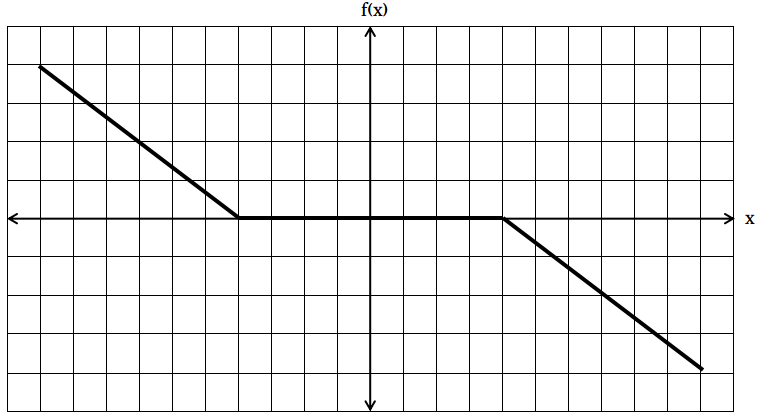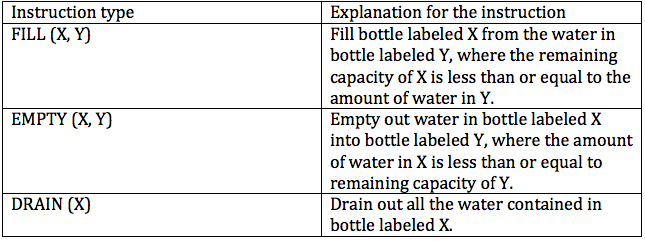# CAT 2000

Instructions

Directions for the next 3 questions:

Given below are three graphs made up of straight-line segments shown as thick lines. In each case choose the answer as:

a) if f(x)=3f(-x)

b) if f(x)= -f(-x)

c) if f(x) = f(-x)

d) if 3f(x) = 6f(-x), for x >= 0

Question 121

Question 122

#Instructions

Directions for the next 2 questions: There are three bottles of water, A, B, C, whose capacities are 5 litres, 3 litres, and 2 litres respectively. For transferring water from one bottle to another and to drain out the bottles, there exists a piping system. The flow through these pipes is computer controlled. The computer that controls the flow through these pipes can be fed with three types of instructions, as explained below:Initially, A is full with water, and B and C are empty.

Question 123

Question 124

# Consider the same sequence of three instructions ‘and the same initial state mentioned above. Three more instructions are added at the end of the above sequence to have A contain 4 litres of water. In this total sequence of six instructions, the fourth one is DRAIN (A). This is the only DRAIN instruction in the entire sequence. At the end of the execution of the above sequence, how much water (in litres) is contained in C?

Instructions

Directions for the next 2 questions:

For a real number x, let

$$f(x) = 1/(1+x),$$ if $$x$$ is non-negative
$$f(x) = 1+x,$$ if $$x$$ is negative

$$f^n(x) = f(f^{n-1}(x)), n = 2, 3.....$$

Question 125

Question 126

# r is an integer 2. Then, what is the value of $$f^{r-1}(-r) + f^r(-r) + f^{r+1}(-r)$$?

Instructions

For the following questions answer them individually

Question 127

Question 128

Question 129

Question 130

OR